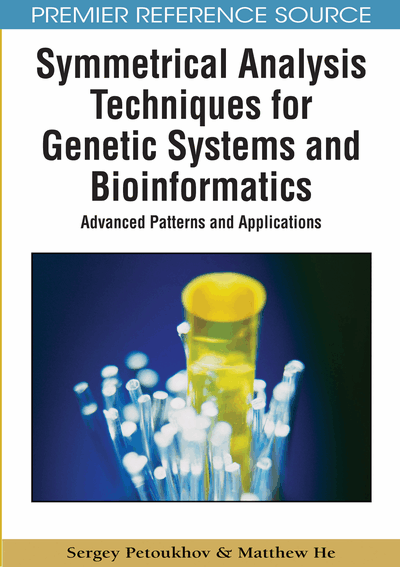# The Genetic Code, Hadamard Matrices, Noise Immunity, and Quantum Computers

Sergey Petoukhov (Russian Academy of Sciences, Russia) and Matthew He (Nova Southeastern University, USA)
DOI: 10.4018/978-1-60566-124-7.ch006
Available
\$37.50
No Current Special Offers

Chapter Preview
Top

## Introduction And Background

We continue to investigate connections of the genetic matrices with matrix formalisms of the theory of discrete signals processing. One of the most famous and the most important kinds of matrices in this theory are the so called Hadamard matrices. These matrices are used also in many other fields due to their advantageous properties: in error-correcting codes such as the Reed-Muller code; in spectral analysis; in multi-channels spectrometers with Hadamard transformations; in quantum computers with Hadamard gates (or logical operators), in quantum mechanics as unitary operators, etc.

Does any natural connection exist between the genetic matrices, which were described in previous chapters, and Hadamard matrices? This question should be investigated especially because a possible positive answer to it may lead to many significant consequences and new thoughts about structures of the genetic code. This chapter demonstrates the existence of such a connection and analyzes some questions related to it.

A huge number of scientific publications are devoted to Hadamard matrices. These matrices give effective opportunities for information processing.

By definition a Hadamard matrix of dimension “n” is the (nxn)-matrix H(n) with elements “+1” and “-1”. It satisfies the condition H(n)*H(n)T = n*In, where H(n)T is the transposed matrix and In is the (nxn)-identity matrix. The Hadamard matrices of dimension 2k are given by the recursive formula H(2k) = H(2)(k) = H(2)⊗H(2k-1) for 2 ≤ kN, where ⊗ denotes the Kronecker (or tensor) product, (k) means the Kronecker exponentiation, k and N are integers, H(2) is demonstrated in Figure 1.

Rows of a Hadamard matrix are mutually orthogonal. It means that every two different rows in a Hadamard matrix represent two perpendicular vectors, a scalar product of which is equal to 0. The element “-1” can be disposed in any of four positions in the Hadamard matrix H(2). Such matrices are used in many fields due to their advantageous properties: in error-correcting codes such as the Reed-Muller code; in spectral analysis and multi-channel spectrometers with Hadamard transformations; in quantum computers with Hadamard gates, etc. It was discovered unexpectedly that Hadamard matrices reflect essential peculiarities of molecular genetic systems (Petoukhov, 2005, 2006, 2008a-d).

Normalized Hadamard (2x2)-matrices are matrices of rotation on 450 or 1350 depending on an arrangement of signs of its individual elements. A Kronecker product of two Hadamard matrices is a Hadamard matrix as well. A permutation of any columns or rows of a Hadamard matrix leads to a new Hadamard matrix.

## Complete Chapter List

Search this Book:
Reset